# AP Calculus BC : Concavity

## Example Questions

### Example Question #1 : Second Derivatives

Over which of the following intervals is the following function concave upward?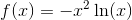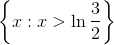f(x) is never concave upward.

{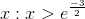}

{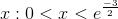}

{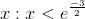}

{}

Explanation:

In order to determine the concavity of a function, we need to examine its second derivative.

First, we will take the derivative of the function by applying the product rule.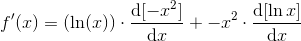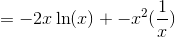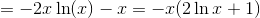We will then take the derivative of the first derivative. Again, we will employ the product rule.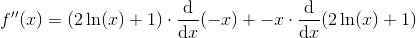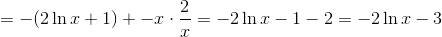A funciton is concave upward whenever its second derivative is positive. Thus, we must find the interval over which f''(x) is positive.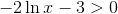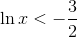This will only be true when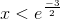. However, keep in mind that the domain of f(x) will include only positive values of x, because lnx is only defined for x > 0.

The answer is {}.

### Example Question #2 : Second Derivatives

Determine the intervals on which the function is concave up: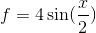on the interval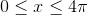The function is never concave up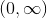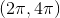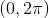Explanation:

To determine the intervals on which the function is concave up, we must find the intervals on which the second derivative of the function is positive.

First, we must find the second derivative of the function: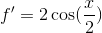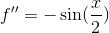The derivatives were found using the following rules: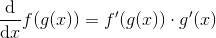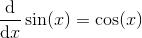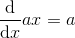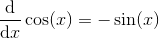Next, we find the values on the given interval for which the second derivative is equal to zero: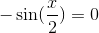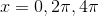We now use these values as bounds for the intervals on which we check the sign of the second derivative: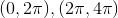Note that at the bounds of the intervals, the second derivative is neither positive nor negative.

Evaluating the sign simply by plugging in any value on the given interval into the second derivative function, we find that on the first interval, the second derivative is negative, while on the second interval, the second derivative is positive. Thus, the function is concave up on the second interval,.

### Example Question #3 : Second Derivatives

Tell whether g(w) is concave up or concave down when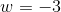. How do you know?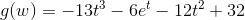Concave up, because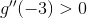Concave up, because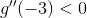Concave down, becauseConcave down, becauseConcave up, becauseExplanation:

Tell whether g(w) is concave up or concave down when. How do you know?To test for concavity, we need to know the sign of our function's second derivative at the given value.

Let's begin by finding the first derivative. To do so, we need to recall the following: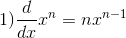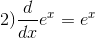Those two rules tell us how to derive functions made up of polynomials and exponential terms.

Apply the rules to get the following.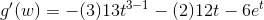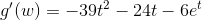Now, do derive again to get the 2nd derivative.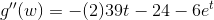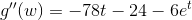Now, we are almost there, but we need to find g"(-3)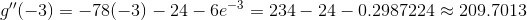So, our second derivative is positive when w=-3. This means our original function is concave up at this point.

Concave up, because### Example Question #4 : Second Derivatives

At which of the following values ofis the function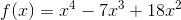concave down?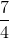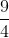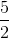Explanation:

The function is concave down wherever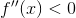, so we compute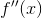and see where it is negative. We have: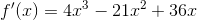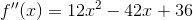(a parabola, opening upwards)

To find whereis negative, we first find its zeros by setting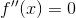: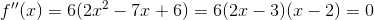,

sowhen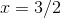or, and we conclude thatis negative (is concave down) between them. That is,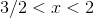. The only answer choice completely inside this interval (not outside or at the endpoints) is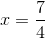.